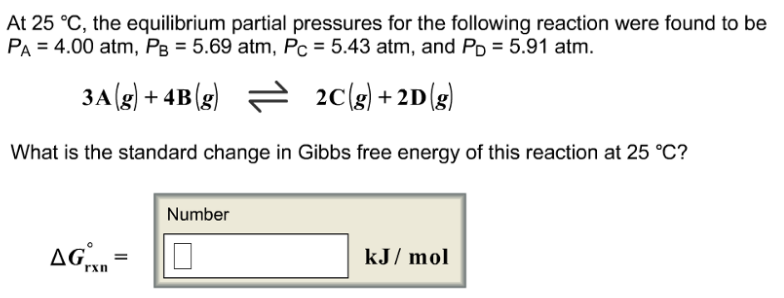# Problem: At 25°C, the equilibrium partial pressures for the following reaction were found to be PA = 4.00 atm, PB = 5.69 atm, PC = 5.43 atm, and PD = 5.91 atm.3A (g) + 4B (g) ⇌ 2C (g) + 2D (g)What is the standard change in Gibbs free energy of this reaction at 25°C?

###### FREE Expert Solution
94% (365 ratings)###### Problem Details

At 25°C, the equilibrium partial pressures for the following reaction were found to be PA = 4.00 atm, PB = 5.69 atm, PC = 5.43 atm, and PD = 5.91 atm.

3A (g) + 4B (g) ⇌ 2C (g) + 2D (g)

What is the standard change in Gibbs free energy of this reaction at 25°C?